Games
Problems
Go Pro!

# Solids of Revolution Volumes

Lesson Plans > Mathematics > Calculus > Integration > Volume

## Slide Show

Click to show full screen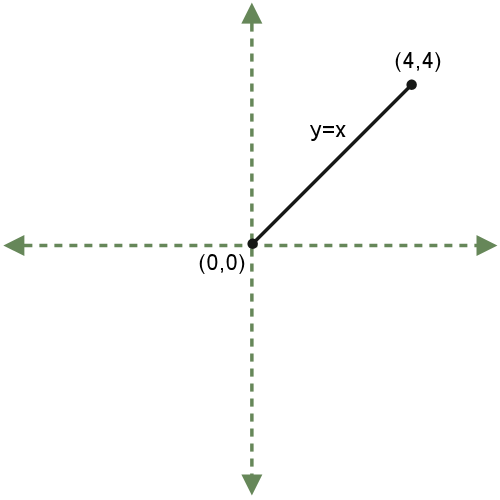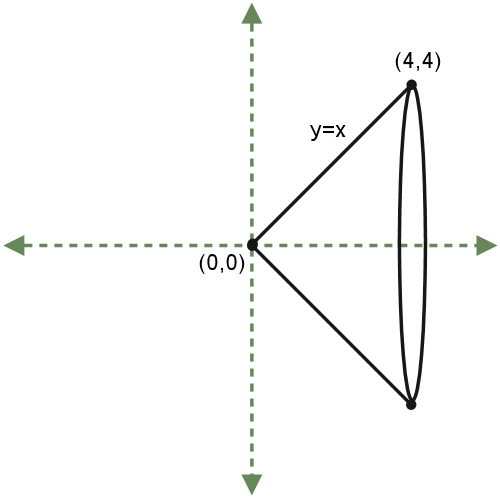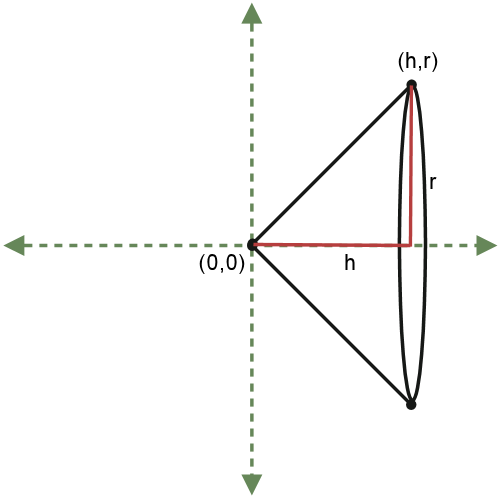## Solids of Revolution VolumesWhen calculus students first learn integration, calculating the volumes of solids of revolutions is a great exercise to spend time on, because students can get impressive results readily. One of my favorite activities is to help students derive the formula for the volume of a cone. I sort of steer them toward this result without telling them (at first) where we're going.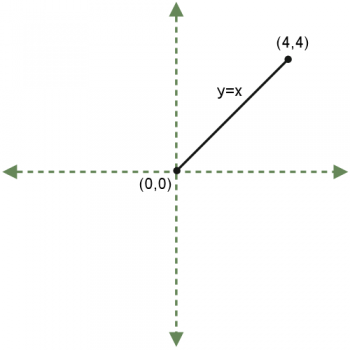I begin by drawing a cartesian plane, and then graphing y = x on the interval [0,4].

"Suppose I took this line segment and spun it around in a third dimension, around the x-axis. What is the three-dimensional shape I would obtain?"

Usually students figure this out; if they don't I draw in the rotation so they can visualize it better.

"It's a cone."

I tell them that this means a cone is a "solid of revolution," which simply means that we can create it by taking a graph of a function and rotating it around the x-axis. "What is the shape of a cross-section of this cone?" The answer, of course, is "a circle."

Now we review what we know about circles; the area of a circle is given by the function A(r) = pr2.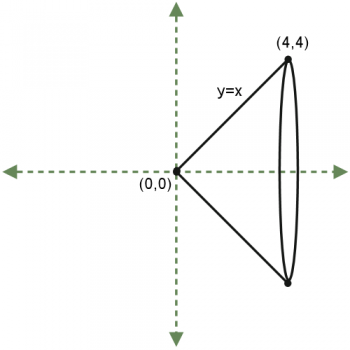This is going to be a helpful function, because to find the volume, we just need to integrate the cross-sectional areas along the given interval.

V(x) =
A(x)dx
=
1
3
pr3 + C

Volume = (
1
3
p(4)3 + C) - (
1
3
p(0)3 + C) =
64
3
p

At this point I say, "This is a wonderful result - we have done a very basic, very simple integration, and we've found the volume of  a cone. But do we really want to do an integration every time we want to find the volume of a cone? Of course not! That's what formulas are for." I check to see if any of my students remember the formula for the volume of a cone: V =
1
3
pr2h. I then show them that using this formula verifies our solution.

Now I spring my question for the day: "Did you ever wonder how in the world mathematicians know that the volume of a cone is V =
1
3
pr2h? Do you think we can prove  that's true?"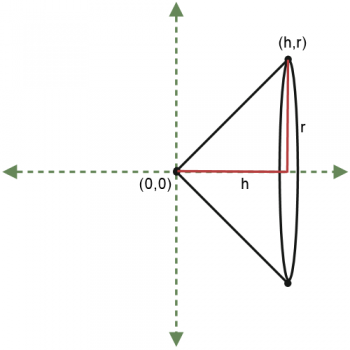I point out that we found the volume of a specific cone (a cone with base radius = 4 and height = 4). If we want to find a general formula, we just need to start with a "general" cone - one in which we don't assign a specific radius and height. In other words, we just use variables r and h to represent these quantities.

The big issue we have here is that instead of starting with an equation of a line, we've started with two segment lengths. This means we don't know the equation of the line (it's not y = x any more!). Without that equation, we can't find the cross-sectional area, which means we can't integrate. So we need to find the equation of the line connecting (0,0) and (h,r).

This is not difficult; the line passes through the origin, so it has 0 as its y-intercept. Its slope is m =
r - 0
h - 0
=
r
h

Thus, the equation is y =
r
h
x.

So the cross-sectional area is given by A(x) = p(
rx
h
)2 =
r2x2p
h2

V(x) =
A(x)dx
=
r2x3p
3h2
+ C

Volume =
h
A(x)dx
0
= (
r2h3p
3h2
+ C) - (
r2(0)3p
3h2
+ C) =
1
3
pr2h

My hope in teaching this lesson is to get students excited to see how some results (like the volume of a cone formula) that they just accepted without questioning in a geometry class, they now have the tools to actually derive for themselves. I tell them that we can use the same techniques to derive formulas for volumes of frustrums and cylinders, which are also solids of revolution.

I spend some time doing additional exercises, like finding the volume when y = x2 is rotated around the x-axis (and also, for good measure, when it is rotated around the y-axis), and then I set them loose on some problems to try on their own.

Lesson by Mr. Twitchell

# Featured Games on This Site

Match color, font, and letter in this strategy game
Trap all the dots in this problem-solving puzzle

# Blogs on This SiteReviews and book lists - books we love!The site administrator fields questions from visitors.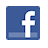Like us on Facebook to get updates about new resources